# Use A Boost Converter To Create An Auto-Dimmable LED Flashlight

## STMicroelectronics L6920 TS951

Jianwen Shao

EDN

Due to their light output and long life, high-brightness LEDs are well-suited for use in flashlights. Typically, the LEDs are driven with a constant current. So when the battery voltage drops, the flashlight just stops working. In some situations, this could be dangerous. It would be nice to have a flashlight that would automatically dim when the battery voltage drops. Just like a traditional flashlight, it would indicate a low-battery condition and would last longer.

Typically, the forward voltage of a white LED is 3 to 5 V. Therefore, if the input voltage drops below 3 V, a boost converter can drive the LED. Figure 1 shows such a boost converter with automatic dimming based on battery voltage.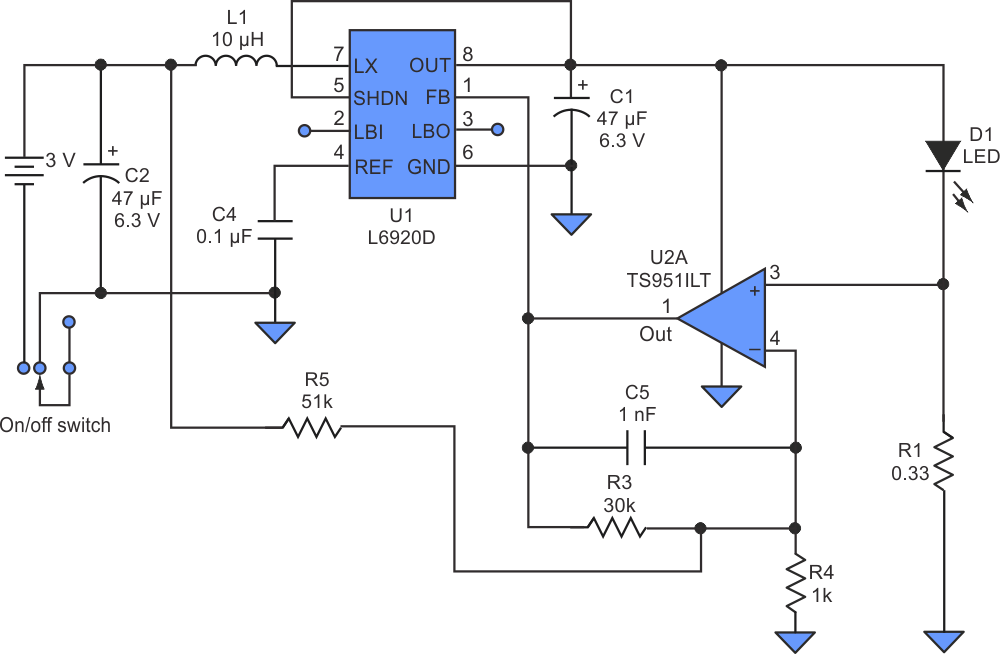Figure 1. Using a high-efficiency step-up controller (U1), designers can create an auto-dimming function for a flashlight that employs high-brightness LEDs.

The L6920D is a high efficiency step-up controller requiring very few external components to convert the battery voltage to the selected output voltage or current. The device will start up at 1 V and operate down to 0.6 V. Its quiescent current is only 10 µA. The device also includes an internal synchronous rectifier implemented with a 120-mW P-channel MOSFET, which replaces the conventional boost diode, to improve the efficiency.

In this circuit, the LED current (ILED) is a function of the battery voltage (VFB). When the battery voltage drops, the LED current drops. In this way, the battery can last longer, and the flashlight will offer dimming characteristics. Assuming VFB = 1.23 V, determined by the L6920D: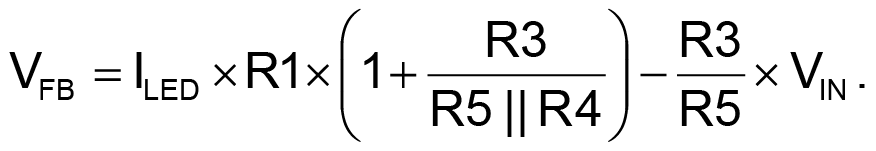If R5 >> R4, then R5||R4 ≈ R4, then: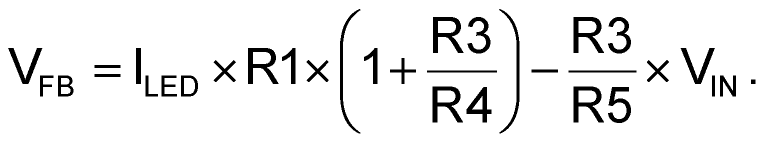Finally: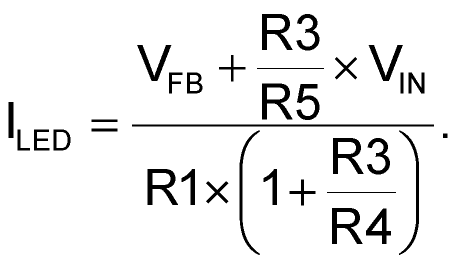Figure 2 shows the resulting LED current calculated using the resistance values in the example circuit.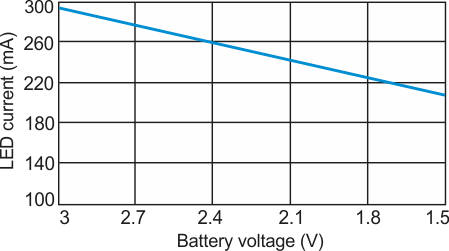Figure 2. For the resistance values in Figure 1, the equation for ILED produced this graph showing how the current drops as the battery voltage decreases.

We compared a constant LED flashlight and this auto-dimming version, using two AAA batteries. Running times were 50 and 90 minutes, respectively. The auto-dimming function nearly doubled the battery life and provided a warning when the battery voltage dropped.

## Materials on the topic

electronicdesign.com